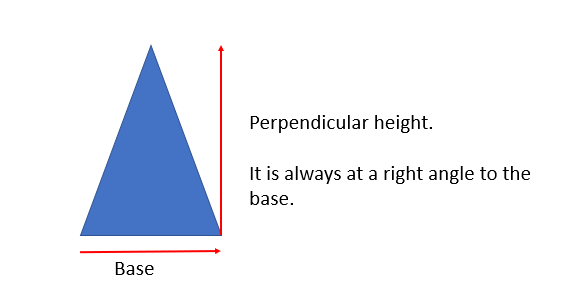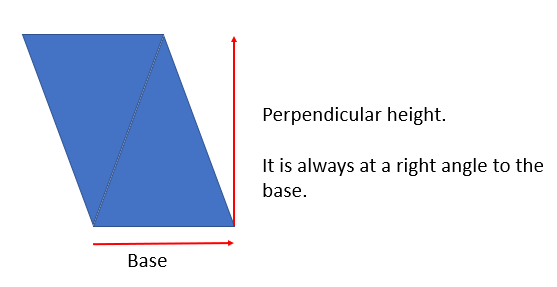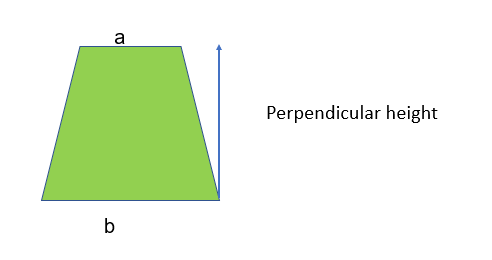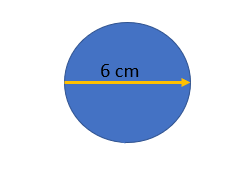# Area of Shapes

## Triangles

To find the area of a triangle use the formula:

1/2 x perpendicular height x base or (perpendicular height x base)/2

1/2 x h x b or (h x b)/2

Remember - always use the perpendicular height!## Parallelograms

The area of a parallelogram is the perpendicular height x base.

(If you double a triangle you get a parallelogram!)## Trapeziums

To find the area of a trapezium:

1. Step 1: Label the parallel lines a and b ( it doesn’t matter which way round)
2. Step 2: Add them together and divide by two ( here you’re finding the average of the lengths)
3. Step 3: Multiply by the height

__Area of a trapezium __= 1/2 (a +b) x hBe prepared to work through this equation backwards.

Eg. A trapezium has an area of 40 cm2 one parallel line with a length of 4 and a height of 8. What is the length of the other parallel line:

Input what we know into the formula:

Area= 1/2 (a+b) x h

40 = 1/2 (4+b) x 8

40 = 4(4+b)

10 = 4 +b

6 = b

## Area of a circle

To work out the area of the circle use this formula:

Area of a circle = π x radius x radius = πr^2

For example:Be careful!! Here we have been given the diameter! This is double the radius, we will have to halve it for our formula to work:

= πr2

= π32

= 9π

= 28.27 (2 decimal places)

The area of a circle is 16π what is the radius?
4
What do the a and b refer to on the formula used to work out the area of a trapezium?
Parallel